VBA DIR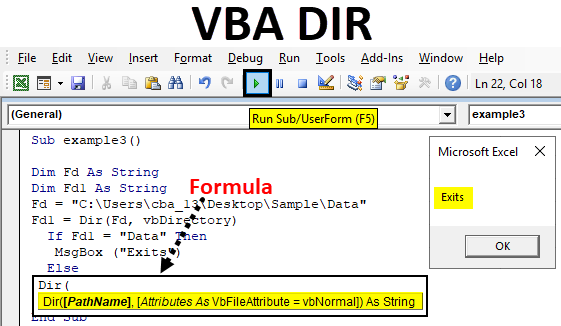Excel VBA DIR Function

Dir is one of the functions available in VBA. Dir Function is for referring Directories in VBA code.
The function that returns the file or directory name that matches with the given attribute or string otherwise returns the first file or folder. In simple words, if we have a folder ABC and inside ABC there is a file XYZ then we can access XYZ file using DIR function in VBA.

Formula For DIR Function in Excel VBA

DIR function has the following syntax in Excel VBA: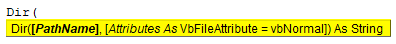It has two parts Pathname and Attributes.

• Pathname: By the name, everyone can understand it is the path of the file where actually the file exists. If we do not input any path in pathname it will return an empty string.
• Attribute: It is an optional argument, we do not use much of this. We may use this when we want to open the file with the below attributes then VBA looks for those files.
 vbArchive Specifies Archives or Backup Files. vbNormal Normal (Default) or no attributes. vbReadOnly read-only files vbSystem System files vbVolume volume label; If you use any other attribute with this one then, volume label is ignored. vbDirectory Directories or Folders. vbHidden hidden files vbAlias File name is an alias

How to Use DIR Function in Excel VBA?

Below are the different examples to use DIR Function in Excel using VBA code.

You can download this VBA DIR Excel Template here – VBA DIR Excel Template

VBA DIR Function – Example #1

In this example, we will see how to access a file name using the DIR function in VBA.

Step 1: Go to the Developers tab and click on Visual Basic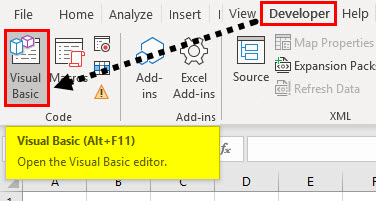Step 2: Open a Module from the Insert menu option as shown below.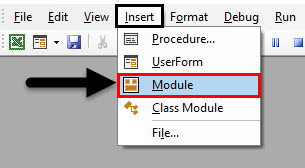Step 3: To start any macro, first we need to give a name to the macro with the keyword ‘sub’ as below.

Code:

Sub myexample1()

End Sub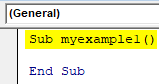Remember we are writing our code in “Module1”  when we input “sub myexample()”, automatically the “End sub” will appear.

Step 4: Define a string by using the keyword “Dim” which refers to dimension. In the below screenshot “mystring” is the string name.

Code:

Sub myexample1()

Dim mystring As String

End Sub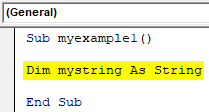Remember whenever you define the names of data types or program names there should not be any space between two words. “Mystring” no space between “my” and “string”.

The file is available in sample folder which is available on the Desktop and the file name is “KT tracker mine”.

C:\Users\cba_13\Desktop\Sample\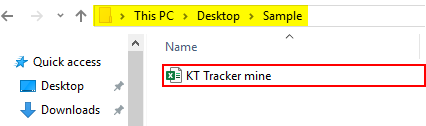Step 5: Now we need to store the file name in “mystring” using Dir function.

Code:

Sub myexample1()

Dim mystring As String
mystring = Dir("C:\Users\cba_13\Desktop\Sample\")

End Sub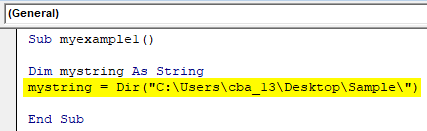In the above screenshot in Dir function, I have given the file path excluding file name. As there is only one file it will return the file name.

Step 6: Now with the above step the file name stored in the string “mystring”. To display the file name, we need to display it through a message box. Create a message box.

Code:

Sub myexample1()

Dim mystring As String
mystring = Dir("C:\Users\cba_13\Desktop\Sample\")
MsgBox (mystring)

End Sub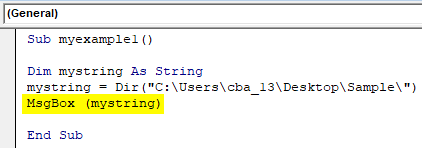We gave the instruction to display the data in  “mystring” through message box, as “mystring” has file name it will display the file name through the message box.

Step 7: Now run the code by clicking on the Play button or by pressing the F5 key.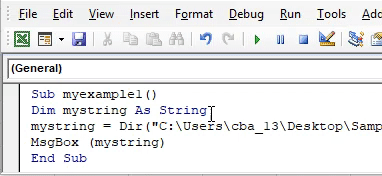VBA DIR Function – Example #2

In this example, we will see how to open a file using the DIR function in Excel VBA.

Step 1: Open the VBA code screen and start by giving the program name as “example2” or any name you wish for.

Code:

Sub example2()

End Sub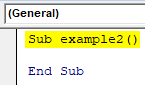Step 2: Define two strings with the names “Foldername” and “Filename”.

Code:

Sub example2()

Dim Foldername As String
Dim Filename As String

End Sub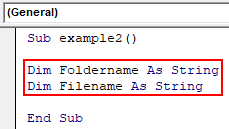Step 3: Now assign the folder path to the folder name.

Code:

Sub example2()

Dim Foldername As String
Dim Filename As String
Foldername = "C:\Users\cba_13\Desktop\Sample\"

End Sub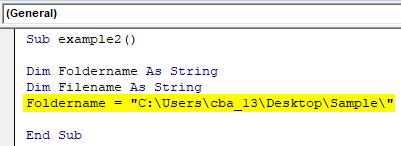Step 4: Assign the file to the “filename” using the Dir function. Here we used the “Foldername” variable because it has the folder path. With the help of ampersand, we added the file name to the path.

Code:

Sub example2()

Dim Foldername As String
Dim Filename As String
Foldername = "C:\Users\cba_13\Desktop\Sample\"
Filename = Dir(Foldername & "KT Tracker mine.xlsx")

End Sub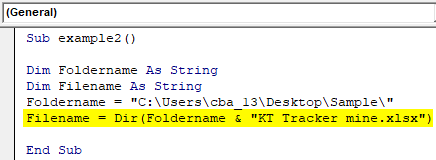Now Dir function returns the file name and it will store in the variable “filename”.

Step 5: In the previous example, we used the message box to see the results. But, in this we want to open the file, so we will use the command “workbooks.open”.

Code:

Sub example2()
Dim Foldername As String
Dim Filename As String
Foldername = "C:\Users\cba_13\Desktop\Sample\"
Filename = Dir(Foldername & "KT Tracker mine.xlsx")
Workbooks.Open Foldername & Filename

End Sub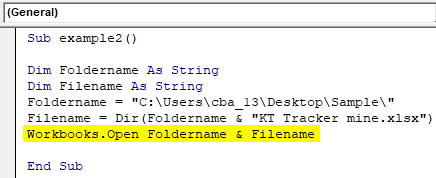Step 6: Run the above code it will open the file available in the folder “C:\Users\cba_13\Desktop\Sample\” with the file name “KT Tracker mine”.

VBA DIR Function – Example #3

Now we will see the program to know whether a folder is available or not. I have the folder “Data” as shown below.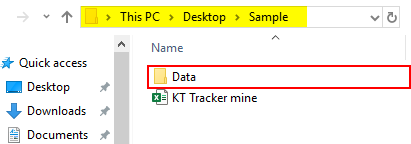We have to check with the help of Dir function whether “Data” folder is available in the path C:\Users\cba_13\Desktop\Sample\.

Step 1: Create a program name and define two strings with the names FD and FD1.

Code:

Sub example3()

Dim Fd As String
Dim Fd1 As String

End Sub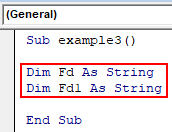Step 2: Assign the folder path to variable “Fd”.

Code:

Sub example3()

Dim Fd As String
Dim Fd1 As String
Fd = "C:\Users\cba_13\Desktop\Sample\Data"

End Sub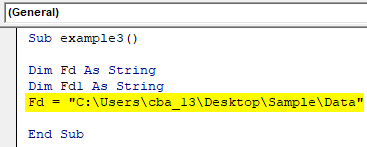Step 3: Now use the Dir function to return the folder name as shown below.

Code:

Sub example3()

Dim Fd As String
Dim Fd1 As String
Fd = "C:\Users\cba_13\Desktop\Sample\Data"
Fd1 = Dir(Fd, vbDirectory)

End Sub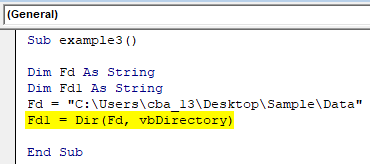The Dir function result should be folder name and it will store in Fd1 string variable. If the “Data” folder is not available in the respective folder, it will not return any string.

Step 4: Now we need to check whether Fd1 has a “Data” folder or not. Use IF condition to check whether Fd1 has string “Data” or not. If it is available, then print the statement as “Exists”.

Code:

Sub example3()

Dim Fd As String
Dim Fd1 As String
Fd = "C:\Users\cba_13\Desktop\Sample\Data"
Fd1 = Dir(Fd, vbDirectory)
If Fd1 = "Data" Then
MsgBox ("Exits")

End Sub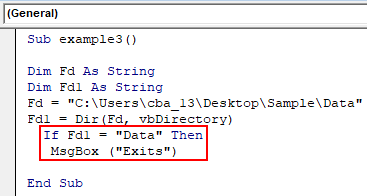Step 5: In case if Fd1 does not match with “Data” folder will print the statement “Not Exists” in else condition.

Code:

Sub example3()

Dim Fd As String
Dim Fd1 As String
Fd = "C:\Users\cba_13\Desktop\Sample\Data"
Fd1 = Dir(Fd, vbDirectory)
If Fd1 = "Data" Then
MsgBox ("Exits")
Else
MsgBox ("Not Exits")

End Sub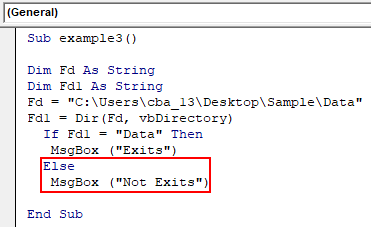Step 6: End the “If” loop as shown in the below screenshot.

Code:

Sub example3()

Dim Fd As String
Dim Fd1 As String
Fd = "C:\Users\cba_13\Desktop\Sample\Data"
Fd1 = Dir(Fd, vbDirectory)
If Fd1 = "Data" Then
MsgBox ("Exits")
Else
MsgBox ("Not Exits")
End If

End Sub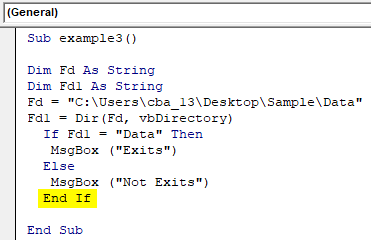Step 7: Run the code by pressing the F5 key or by clicking on the Play button to check whether the folder is available or not. If the folder “Data” is available in that folder, then it will return the message box with the message “Exists” as below.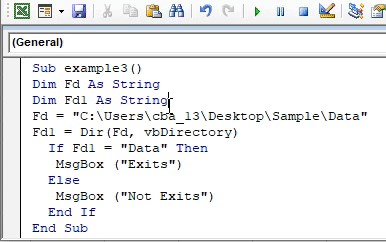Step 8: Just change the name of the folder as Data1.

Sub example3()

Dim Fd As String
Dim Fd1 As String
Fd = "C:\Users\cba_13\Desktop\Sample\Data1"
Fd1 = Dir(Fd, vbDirectory)
If Fd1 = "Data" Then
MsgBox ("Exits")
Else
MsgBox ("Not Exits")
End If

End Sub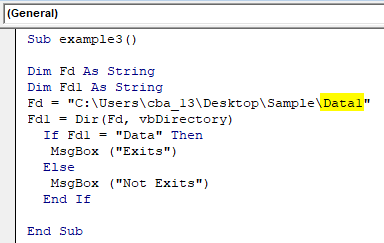Step 9: Run the code again. Now the result is “Not exists” as shown below.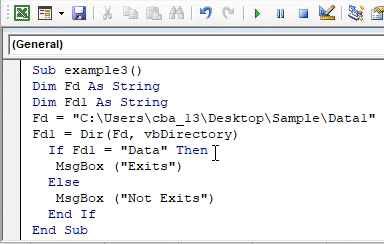Things to Remember

• The arguments in the Dir function are optional.
• If we want hidden files, folders or different type of directories then mention your requirement in the second argument.
• Use “\” at the end of the folder name while giving in Dir function to go into the folder.
• If we want to call or open multiple files we can use the wild card character “*” or “?”.

Recommended Articles

This is a guide to Vba Dir Function. Here we discuss how to use DIR Function in Excel VBA along with some practical examples and downloadable excel template. You can also go through our other suggested articles –

1. VBA Workbook
2. INDIRECT Function in Excel
3. VBA Count
4. Excel XOR Function

The post VBA DIR appeared first on EDUCBA.

This post first appeared on Best Online Training & Video Courses | EduCBA, please read the originial post: here

Subscribe to Best Online Training & Video Courses | Educba

Get updates delivered right to your inbox!

×